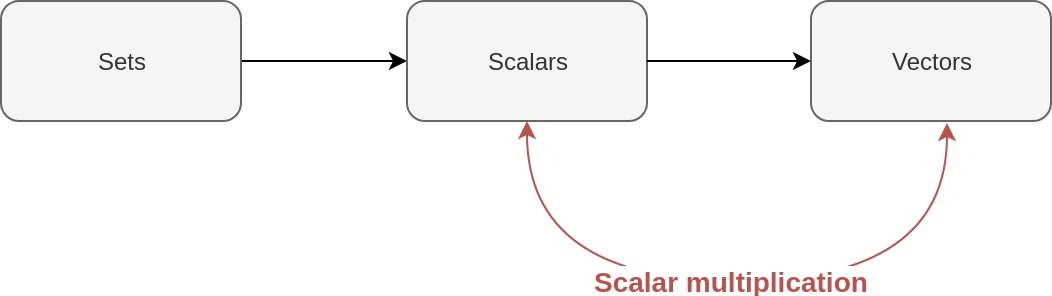# Deep (learning) like Jacques Cousteau - Part 4 - Scalar multiplication

(TL;DR: Multiply a vector by a scalar one element at a time.)

LaTeX and MathJax warning for those viewing my feed: please view directly on website!We build, we stack, we multiply

Nate Dogg from ‘Multiply’ by Xzibit

Last time, we learnt about vectors. Before that, we learnt about scalars. What happens when we multiply a vector by a scalar?(I don’t know where I’m going with this diagram…but bear with me!)

# Today’s topic: Multiplying vectors by scalars

Let’s use our vector $\boldsymbol{x}$ from last time.

Let’s pick a scalar to multiply it by. I like the number two, so let’s multiply it by two!

To evaluate this, we perform scalar multiplication. That is, we multiply each element of our vector by our scalar. Easy!

More generally, if our vector $\boldsymbol{x}$ contains $n$ elements and we multiply it by some scalar $c \in \mathbb{R}$, we get:

## How can we perform scalar multiplication in R?

This is easy. It’s what R does by default.

Let’s define our vector, x.

x <- c(1, 2, 3)
print(x)

##  1 2 3


Let’s define our scalar, c.

c <- 2
print(c)

##  2


Now, let’s multiply our vector by our scalar.

c * x

##  2 4 6


Boom! The power of vectorisation!

## How does type coercion affect scalar multiplication?

The comments we made in an earlier post about type coercion apply here. Let’s define x as an integer vector.

x <- c(1L, 2L, 3L)
class(x)

##  "integer"


Our scalar c may also look like an integer, but it has been stored as a numeric type, which is our proxy for real numbers.

print(c)

##  2

class(c)

##  "numeric"


So when we multiply a numeric type by our integer vector, we get a result in the more general numeric type!

class(c * x)

##  "numeric"


# Conclusion

To multiply a vector by a scalar, simply multiply each element of the vector by the scalar. This is pretty easy, isn’t it?

Let’s learn how to add two vectors before we cover dot products. Only then can we enter the matrix!

Justin

Categories:

Updated: